# Reduction Process

All variables in the resonant layer are assumed to be functions of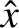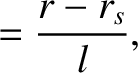(4.35)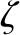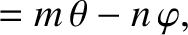(4.36)

and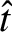, only. Here,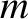and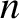are the poloidal and toroidal mode numbers, respectively, of the tearing mode,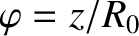is a simulated toroidal angle, andis the simulated major radius of the plasma. (See Chapter 3.)

Let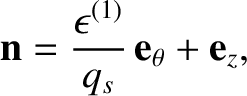(4.37)

where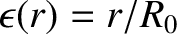and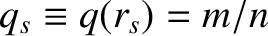. Here,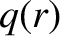is the safety factor profile. [See Equation (3.2).] Moreover, the superscript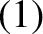indicates a quantity that is first order in our ordering scheme. [Zeroth order terms are left without superscripts, whereas second order terms are given the superscript (2).]

It follows that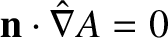(4.38)

for any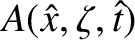. Furthermore,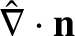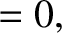(4.39)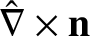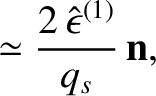(4.40)

where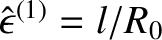.

We can automatically satisfy Equation (4.32) by writing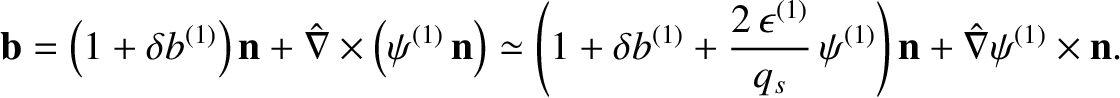(4.41)

It follows that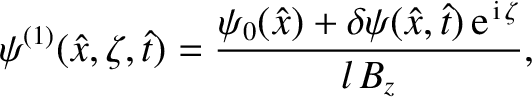(4.42)

where [see Equations (3.1) and (3.2)]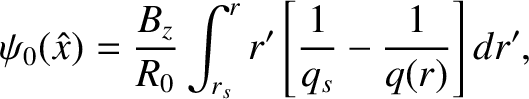(4.43)

and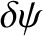is defined in Equation (3.20). Note that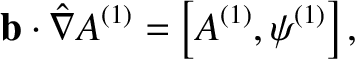(4.44)

where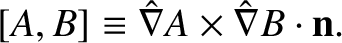(4.45)

Equations (4.33) and (4.41) give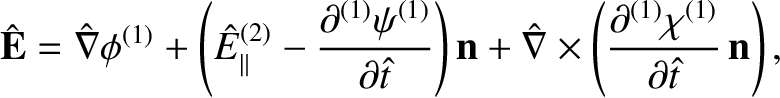(4.46)

where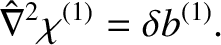(4.47)

Here, we have explicitly separated out the time dependent, spatially uniform part of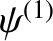which generates the (normalized) inductive electric field,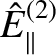, that is responsible for maintaining the equilibrium parallel current density in the inner region against ohmic decay.

Equations (4.34) and (4.41) yield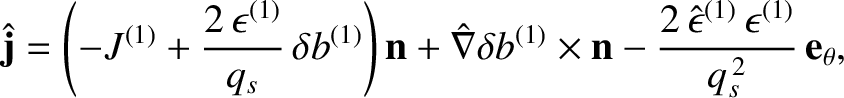(4.48)

where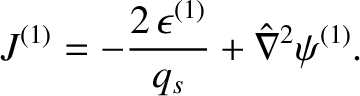(4.49)

Let us write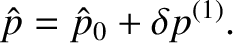(4.50)

Note that the ordering of the plasma pressure adopted here is somewhat different to that adopted in Section 3.3. In fact, in Section 3.3,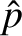was assumed to be second order. Here, we are assuming thathas a spatially constant component that is zeroth order, and a spatially varying component that is first order. This high pressure ordering is merely an artifice to aid the extraction of the compressible-Alfvén (i.e., fast magnetosonic) wave from the system of equations [3,4]. In fact, the ordering ensures that the compressible-Alfvén wave has a substantially different phase velocity than the shear-Alfvén and slow magnetosonic waves .

Equations (4.28)–(4.31), (4.41), (4.46), and (4.50) yield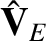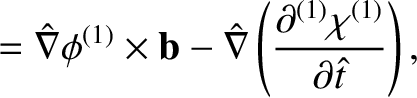(4.51)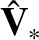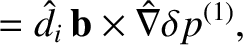(4.52)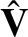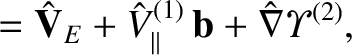(4.53)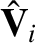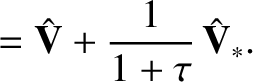(4.54)

Here, the additional factor involving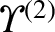in Equation (4.53) is needed because the plasma flow is slightly compressible. In fact, the velocity fields,,, andall have normalized divergences that are second order.

Evaluating the normalized drift-MHD fluid equations, (4.25)–(4.27), up to second order, we obtain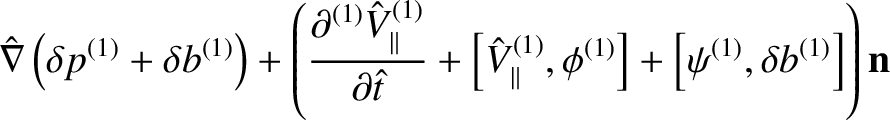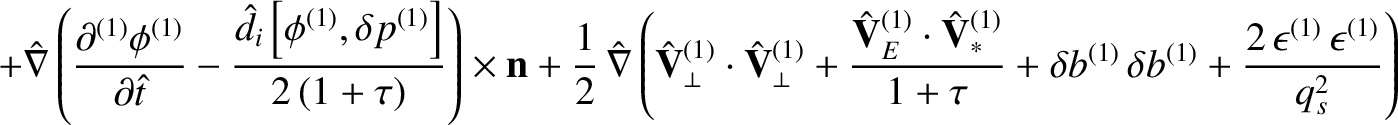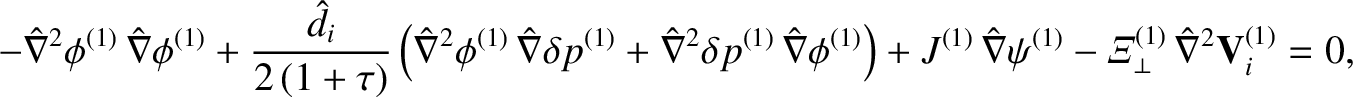(4.55)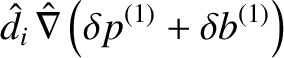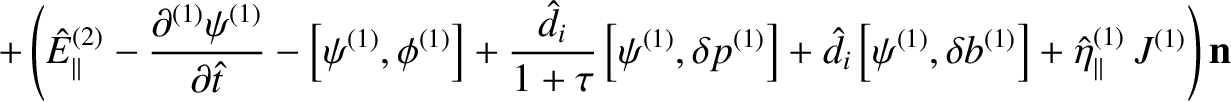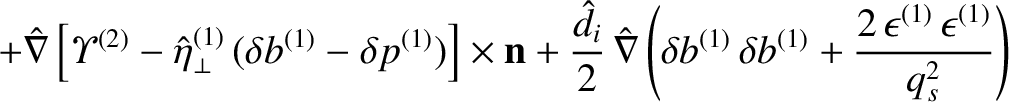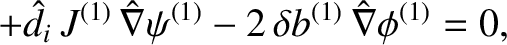(4.56)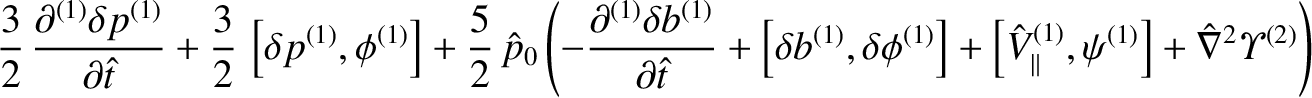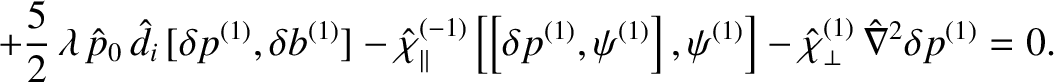(4.57)

To first order, Equations (4.55) and (4.56) both yield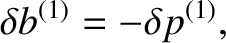(4.58)

which is simply an expression of lowest-order equilibrium force balance [3,7].

Taking the scalar product of Equation (4.55) with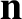annihilates the first-order terms, leaving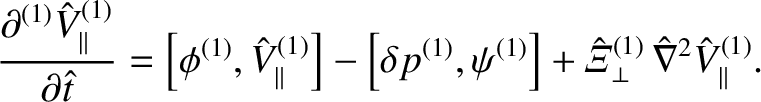(4.59)

Moreover, taking the scalar product of Equation (4.56) withannihilates the first-order terms, leaving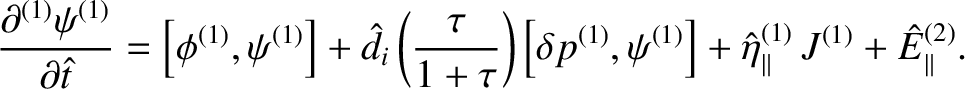(4.60)

Furthermore, taking the scalar product of the curl of Equation (4.55) withannihilates the first-order terms, leaving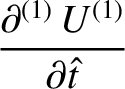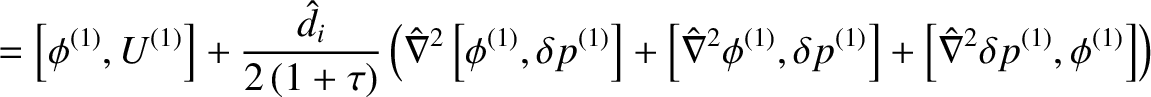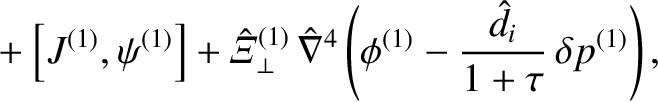(4.61)

where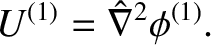(4.62)

Finally, taking the scalar product of the curl of Equation (4.56) withannihilates the first-order terms, leaving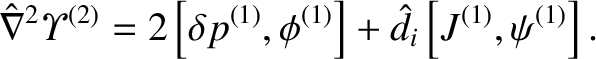(4.63)

The previous equation can be combined with Equations (4.57) and (4.58) to give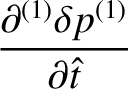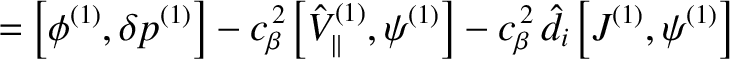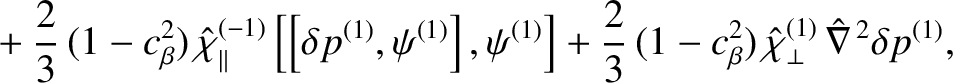(4.64)

where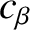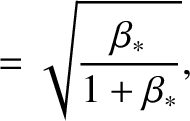(4.65)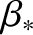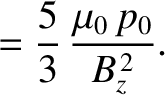(4.66)